﻿ The Excel IF function
You are on the Home/Publications & Training/Case Studies/The Excel IF functionShare Your

# The role of the IF function in an Excel formula

This might seem to be a strange claim but the IF function is one of the less understood functions in Excel.  The key reason for this, I suspect, is that most people, particularly those who develop software, think of the IF as a sort of a statement – and, a statement, of course, is an executable that yields a “final” result.   So, we expect that if a condition is satisfied the code will do one thing and if the condition is not satisfied do something else.  However, an Excel formula doesn’t do anything that visibly changes the environment.  Instead, it computes, i.e., calculates, a result of some sort.  Consequently, and strictly speaking, in Excel we don’t have an IF statement.  What we do have is an IF function.  For the developer, this would be the equivalent of an IIf function in Visual Basic – IIf(condition, result-if-true, result-if-false) – or the ternary conditional in C (or JavaScript) – condition? result-if-true: result-if-false

The IF function is like any other function in that the value it returns can be used anywhere where you would use an item of the type returned by the IF function.  So, in a formula that has a number one can replace that number with an IF function that returns a number.  Similarly, if one has a formula that contains text, one can replace that text with an IF function that returns a text result.  And, for those interested in array formulas, yes, the IF function is ‘array aware’ though a discussion of the IF function in an array formula is left for another Excel tip.

Example 1: Every so often I get a coupon from an office supply store (such as Office Depot or Staples) that offers a fixed discount if my purchase totals more than a specified amount.  A recent coupon offered \$20 off if I spent more than \$100.  Suppose we had this information in an Excel spreadsheet: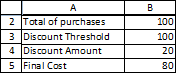Then, we can name those cells as below:

 Total_of_purchases =Sheet3!\$B\$2 Discount_Amount =Sheet3!\$B\$4 Discount_Threshold =Sheet3!\$B\$3 Final_Cost =Sheet3!\$B\$5

With the above set up, one way to calculate the final purchase cost is to use a IF to calculate the final result.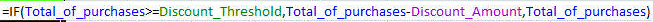But, that is not the only way to think of the final cost.  In fact, one can interpret the incentive as “take \$20 off if your total is over \$100 else take \$0 off.”  So, one can create an Excel formula such as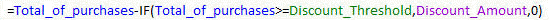In a lot of ways this is a more natural way to construct more complex results.

Example 2: In our second example, suppose the office supply store offered a more complex discount structure – and this is actually the kind of offer I do get once in a while.  “Take \$30 off if you spend \$150 or more; take \$20 off if you spend \$100 or more.”  If we had used the typical way of using the IF function, we would get something that quickly gets messy.On the other hand, treating the IF function as a function yields the structure below, which is a lot easier to manage than the above.It is also easier to extend the IF clauses if we had yet another threshold and discount to incorporate

By now it should be apparent that if there is a function in a formula, one can replace that function with an IF function.  Similarly, one can replace a cell reference or a constant with an IF function!

Next, we look at two more examples to illustrate the role of the IF function.  The first one reinforces the idea of how an IF function can be embedded in place of any number in a formula.  We will build it in three steps.  The next, and final, example is a much more complex formula, which, too, we will build in steps of increasing complexity.

Example 3: As a result of a recent acquisition, a company has two groups of hourly employees.  Those in Group 1 will receive an increase in the hourly wage from \$12 to \$15 effective January 12, 2008.  Those in Group 2 will receive the same increase except that it will be effective June 12, 2008.

We will start with a worksheet model that returns how much to pay someone for a day’s work.  If this person works on a weekday, the pay is the regular hourly wage multiplied by the hours worked.  If, however, this person works on a weekend (Saturday or Sunday), the hourly wage is multiplied by 1.5.  We will start with the following layout: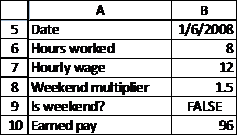The various cells are named as below:

 Earned_pay =Sheet1!\$B\$10 Hourly_wage =Sheet1!\$B\$7 Hours_worked =Sheet1!\$B\$6 Is_weekend? =Sheet1!\$B\$9 Weekend_multiplier =Sheet1!\$B\$8

In the traditional way of thinking, the Earned Pay calculation will do one thing (calculate the weekend pay) or the other (calculate the weekday pay).  Consequently, we would use the formula =IF(Is_weekend?,Hours_worked*Hourly_wage*Weekend_multiplier,Hours_worked*Hourly_wage*1)

However, this is an instance where one is not leveraging the fact that Excel supports the IF function and not the IF statement.  A function can return any value not just a "final" result.  So, we could use

=Hours_worked*Hourly_wage*IF(Is_weekend?,Weekend_multiplier,1)

What’s the advantage of this formula?  In a lot of ways this is how we think of the problem, i.e., we multiply the hourly wage by the weekend multiplier if the workday falls on a weekend.  From the perspective of the workbook modeler (or reviewer) the formula contains only a single reference to the various predecessors.  This makes the formula easier to read, understand, and maintain.

But, this is not all there is to the IF function.  In the above formula we replaced the "wage multiplier" by an IF function.����������� In our next example we will look at a more advanced problem.

Example 4:  This builds on example 3.  The wages earned by the company’s workers are also based on overtime.  If the person works more than 8 hours on a weekday or more than 4 hours on a weekend then the extra hours earn a 100% premium.  So, we get the following table for calculating the daily earnings

 Hours worked below the overtime threshold Hours worked above the overtime threshold Weekend Hourly wage * hours worked * weekend multiplier Hourly wage * hours worked over threshold * weekend multiplier * overtime multiplier Weekday Hourly wage * hours worked Hourly wage * hours worked over threshold * overtime multiplier

As the first step, we include this information in the worksheet.We also name these cells

 Weekday_overtime_threshold =Sheet1!\$B\$11 Weekend_overtime_threshold =Sheet1!\$B\$12 Overtime_multiplier =Sheet1!\$B\$13

Next, we calculate the weekend earnings.  In the formula below the MIN() term calculates the hours worked below the overtime threshold.  If the person worked more than the overtime threshold it returns the threshold itself (4 in our example).  These many hours earn only the weekend multiplier.  The MAX() term, on the other hand, computes the number of hours worked that are above the overtime threshold.  These hours earn the weekend multiplier times the overtime multiplier.We can, of course, factor out the common terms in the equation to get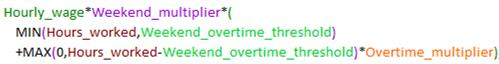Next, we will incorporate the weekday information.  The traditional approach would use an over-arching IF: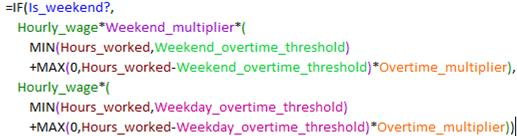To those accustomed to thinking of conditionals as statements, the above probably makes sense.  But, it is far from the most effective way of writing the formula.  It doesn’t reflect the structure of the problem, wherein the definition of overtime-vs.-regular pay was independent of day-of-the-week; what was different was the threshold for what constituted overtime.  Also, the formula is repetitive and will require additional work and testing when we have to update the formula.

Instead, we leverage the fact that the IF is a function and not a statement.  This way of thinking is also much closer to the original problem description.  In our problem description, we had the same structure for the overtime analysis irrespective of whether it was a weekday or a weekend.  The only difference was the threshold used for defining when overtime started.  And, of course, the wage multiplier is 1 for a weekday and Weekend_multiplier for a weekend.

Remember we can use the IF function anywhere we have a constant.  So, we go back to the weekend formula and replace the appropriate constants with corresponding IF constructs: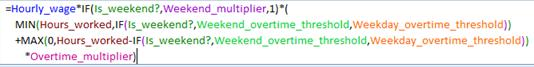Note that the above formula is structurally closer to the problem description.  It also avoids a repetitive description of nearly identical components for weekday or weekend.  This can be very important since, if we had more detailed requirements (say, there was one wage multiplier for a weekday, a second one for a Saturday, and third one for a Sunday), the formula complexity would grow a lot less with this approach as compared with the traditional one, which would simply become three times as complex.

In summary, one should leverage the fact that Excel supports the IF function and not the IF statement.  This will allow one to construct formulas that are simpler to read and easier to maintain.  One should also remain aware of the fact that Excel calculates only one of the true or false components (depending on whether the conditional is true or false), and not both.

 In terms of performance Excel’s IF function is like the C ternary construct in that only one of the 2 true/false clauses is evaluated.  This is unlike the Visual Basic IIf function in which both the true and the false parts are executed every time.

 Using the IF as a function results in a formula structure that lets us see that the multiple discount model is better addressed with another Excel function, making the formula IF-free.  Given the multiple discounts, we could actually put the information in a table and use the VLOOKUP function.  Suppose we put the thresholds and discounts in a table as below and name the table:

 Discount_Table =Sheet3!\$F\$8:\$G\$10

Now, our formula becomes the simple

 The reader should be comfortable with how the MAX function returns the correct result.  If the person works fewer hours than the overtime threshold, Hours_worked – Weekend_overtime_threshold will be negative.  Then, MAX(0, negative-value) will be zero.  If, on the other hand, the person worked more than the overtime threshold, the Hours_worked – Weekend_overtime_threshold yields the number of hours beyond the threshold that the person worked.